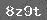# 口算乘法习题精选

1. 填空题2. 在○里填上>，< 或=

①23×40○24×30　　②2700×3○900×9

③78×50○79×50　　④1200×7○430×20

3. 计算

19×3=　　15×5=　　13×6=

230×4=　　26×5=　　250×4=

33×4=　　170×7=　　27×3=

4. 计算题

32×30=　　40×15=　　60×12=

23×30=　　30×50=

5. 240的4倍是多少？

### 相关推荐

 留言与评论（共有 0 条评论）

 验证码：